# Members' research interests

Please read the group core members’ research interest below and for any additional details please visit their PWPs. For the research interest of others (supporting members) please visit their PWPs by clicking their entry on People page.

### Professor Damiano Brigo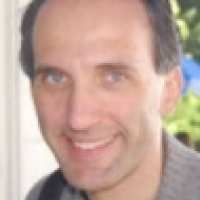Damiano's research in stochastic analysis started with the differential geometric approach to statistics and its use in stochastic dynamical systems, and stochastic filtering in particular, where he co-originated the Projection Filter approach to filtering in the nineties. Research is still active on approximate finite dimensional filters that are obtained by projecting the infinite dimensional stochastic PDE of the filtering problem onto a finite dimensional manifold of probability densities according to a variety of metrics and manifolds. Analytical and numerical studies of the obtained approximations are considered.

Damiano's main interests are in mathematical finance and in particular concern derivatives valuation and hedging, funding costs and BSDEs, counterparty risk pricing and collateral modelling, and the new holistic and non-linear modelling approach emerging from the above points. Further interests include stochastic models for commodities and inflation, dependence dynamics, characterization of self-chaining arrival times, optimal execution and algorithmic trading, liquidity risk measurement and risk measurement in general.

### Dr Thomas Cass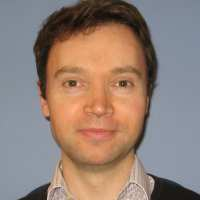Thomas' research comprises both classical areas of Stochastic Analysis such as Malliavin Calculus and newly-emerging disciplines such as Rough Path Theory. He is also interested in the ways in which these mathematical insights can spur developments in numerical simulation in Mathematical Finance. More specifically, some of his interests include:

- The theory of Rough Differential Equations (RDEs), both deterministic and stochastic; the general properties of RDEs driven by Gaussian noise and generalisations of Hoermander's Theorem to the existence and smoothness of the laws of such  processes.
- The use of Malliavin Calculus and cubature methodologies for developing  efficient Monte Carlo based pricing methods in Mathematical Finance.
- Rough Path Theory to understand models of interacting agents with individual preferences. Rough Paths in a geometric setting; Rough Paths on manifolds.

### Dr Ajay Chandra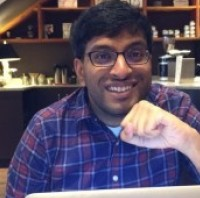Ajay's research interests focus on statistical mechanics, quantum field theory, and stochastic processes. He is particularly interested in critical phenomena in equilibrium statistical mechanics, the construction of quantum fields, and renormalization and behavior of stochastic partial differential equations.

### Professor Dan Crisan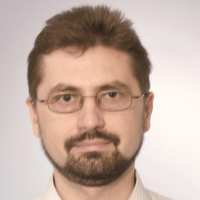Dan’s research lies in the area of the theoretical analysis of stochastic dynamical systems and their numerical approximations grouped into four classes:

- Stochastic partial differential equations, in particular the Zakai and the Kushner Stratonovitch equation. These stochastic PDEs govern the evolution of the solution of the continuous time stochastic filtering problem.

- Partially observed Markov chains. These dynamical systems are related to the discrete time filtering problem. My results cover necessary and sufficient for the convergence of particle approximation to the solution of the filtering problem, optimal algorithms and the stability of the partially observed Markov chains.

- Stochastic differential equations, in particular equations perturbed by degenerate noise and their applications to option pricing.

- Forward-backward stochastic differential equations and their applications to nonlinear option pricing.

General research interests: Nonlinear Filtering, FBSDEs, Mathematical Finance, Particle Approximations, Malliavin Calculus, Stochastic Partial Differential Equations

### Professor Axel Gandy- Statistics in Finance/Business

- Computational Statistics, especially adaptive algorithms

- Survival Analysis/Event History Analysis

- Reliability

### Professor Martin Hairer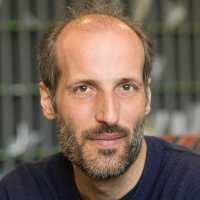My main research interest is the study of stochastic partial differential equations (SPDEs). Problems in this area revolve around the study of their long-time behaviour, the development of the theory of regularity structures, the question of universality of crossover regimes, etc.

Other research interests of mine include nonequlibrium statistical mechanics, stochastic differential equations, stochastic processes with memory, and the general theory of Markov processes.

### Dr Antoine (Jack) Jacquier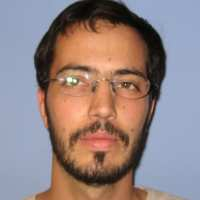Antoine's research mainly focuses on the study of the asymptotic behaviour of stochastic processes.

He has in particular tackled the following issues:

Closed-form representation of the large-time behaviour of affine processes;

Small-noise expansions of densities of projected diffusion processes;

Use of asymptotic methods for robust pricing and calibration of financial derivatives;

General research interests: Large deviations, Implied vo latility, Asymptotic methods, Laplace methods on Wiener space, Mathematical Finance

### Dr Nikolas Kantas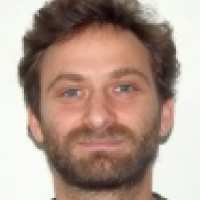Nikolas's research interests are concerned with using simulation based methods for inference and optimisation problems. He focuses mainly on Sequential Monte Carlo and Markov Chain Monte Carlo techniques when applied as computational methods for:

- non-linear filtering

- estimation of model parameters for general state space models

- stochastic optimal control

- rare events estimation

- inverse problems and data assimilation

### Professor Xue-Mei Li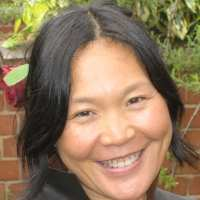Xue-Mei works with stochastic  equations and stochastic dynamical systems and is specially enthusiastic about stochastic analysis on geometric spaces and in infinite dimensional spaces,  the geometry of diffusion processes and of the stochastic dynamical systems, and lately  concrete stochastic models. After solving the open problem on the existence of a global stochastic flow for stochastic differential equations on non-compact manifolds, Xue-Mei uses SDEs as a tool to study the solutions /the fundamental solutions of second order parabolic equations and to study the measures they determined on the space of paths.

Xue-Mei’s research focuses on  the following:

- Stochastic averaging, singular perturbation problems, perturbations to conservation laws and to stochastic dynamical systems,  complex reduction and multi-scale analysis;

- Second order differential operators on manifolds,  their intrinsic geometries, and the geometry of the associated Markov processes;

- Fundamental properties of stochastic flows and stochastic differential equations;

- Malliavin calculus and analysis of infinite dimensional spaces;

- Estimates for the solutions of second order parabolic equations and their derivatives;

- Probability measures on the space of paths and the space of loops;

- Malliavin Calculus;

- Hypo-elliptic operators.

### Professor Johannes Muhle-Karbe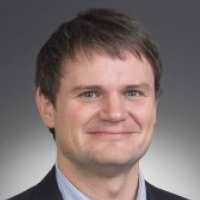Johannes works on the interface of stochastic calculus, optimal control and their application to finance.

For example, he studies limit theorems that reveal the impact of market frictions such as transaction costs, model uncertainty, asymmetric information, or price jumps. In particular, one strand of recent research focuses on the forward-backward equations describing the equilibrium dynamics of illiquid assets.

### Dr Eyal NeumannEyal is a Research Associate at the Department of Mathematics at Imperial College. His research interests are in the area of interacting particle systems, stochastic partial differential equations and mathematical finance. His main focus is on existence and uniqueness questions for stochastic PDEs with Hölder continuous noise coefficients, phase transitions of interacting branching particle systems and singular stochastic control problems which arise from optimal trading.

### Dr Mikko PakkanenMikko's research involves applied probability and stochastic processes, with applications to statistical methods and mathematical finance. In particular, he is interested in:

- Mathematical Finance: market microstructure, modelling of limit order books, stochastic volatility models, transaction costs.

- Probability: ambit stochastics, central and non-central limit theorems, discretisation of stochastic processes, random fields, support theorems.

- Statistics: realised volatility, statistical inference for stochastic processes and random fields.

### Professor Greg Pavliotis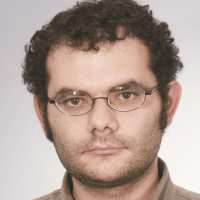Greg's research lies in the areas of stochastic processes and statistical mechanics. Currently he is working on the following problems:

• analysis and numerics for stochastic differential equations - Long time asymptotics for hypoelliptic diffusions, amplitude equations for stochastic PDEs, averaging/homogenization for SDEs, numerical analysis for  Stochastic PDEs with multiple scales,

• statistical inference for diffusion processes -Parametric and non-parametric maximum likelihood estimation for multiscale diffusions, Markov Chain Monte Carlo for nonreversible diffusions,

• statistical mechanics - Transport and kinetic theory,  non-equilibrium statistical mechanics, noise-induced transitions, stochastic modeling of Brownian motors.

### Dr Pierre-Francois Rodriguez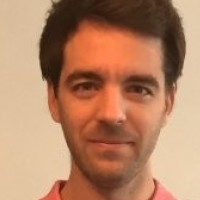Dr Rodriguez's main research area is probability theory, with a special interest in problems related to physics, in particular problems concerning phase transitions and random media.

### Professor Almut Veraart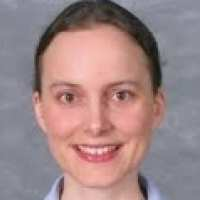Almut's research interests are in statistical methods for stochastic processes, with particular focus on:

• Inference for semimartingales, in particular asymptotic theory based on high frequency data.

• Continuous time modelling of time series, in particular stochastic volatility models.

• Ambit stochastics: Theory of ambit fields and ambit processes with applications to tempo--spatial modelling.

• Applications to Finance, in particular modelling the dynamics of energy prices.

General research interests: Statistica l meth ods for stochastic processes, financial econometrics, applied probability, mathematical finance.